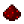# bit.blshiftFunction bit.blshift Shifts a number left by a specified number of bits Syntax bit.blshift(number n, number bits) Returns number the value of n shifted left by bits bits, which is equivalent to n×2bits Part of ComputerCraft API bitExample Shift the number 18 (10010) left by 2 bits, yielding 72 (1001000) Code ```print(bit.blshift(18, 2)) ``` Output 72